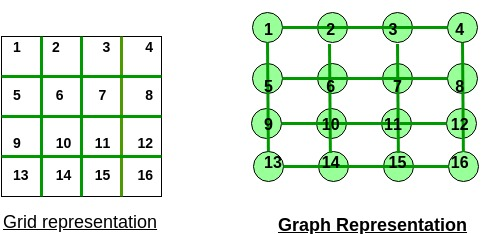# Cost of painting n * m grid

• Last Updated : 29 Apr, 2021

Given two integers n and m which are the dimensions of a grid. The task is to find the cost of painting the grid cell by cell where the cost of painting a cell is equal to the number of painted cells adjacent to it. Any cell can be painted at any time if it hasn’t been painted already, minimize the cost of painting the grid.
Examples:

Input: n = 1, m = 1
Output:
A single cell with no adjacent cells can be painted free of cost
Input: n = 1, m = 2
Output:
1st cell will be painted free of cost but the cost of painting the second cell will be 1 as there is 1 adjacent cell to it which is painted

Attention reader! All those who say programming isn't for kids, just haven't met the right mentors yet. Join the  Demo Class for First Step to Coding Coursespecifically designed for students of class 8 to 12.

The students will get to learn more about the world of programming in these free classes which will definitely help them in making a wise career choice in the future.

A simple solution is to generate all possible ways of painting the grid with n * m cells and that is n * m! calculate the cost for each one of them.
Time Complexity: O(n * m!)
Efficient Approach: Understand the coloring process in this graph instead of the grid. The coloring of a cell is therefore equal to the coloring of a vertex of the graph. The cost obtained for the painting of a cell is equal to the number of colored neighbors of the cell. This means that the cost obtained will be the number of edges between the current cell and the adjacent colored cells.The crucial point is to note that after the end of coloring of all the cells, all the edges of the graph will be marked.
An interesting fact is that the edges of the graph are marked only once. This is because an edge connects two cells. We mark the cell when both cells are painted. We are not allowed to paint a cell more than once, which ensures that we only mark each cell once.
Thus, whatever the order in which you color the cells, the number of marked edges remain the same, so the cost will be the same.
And for given grid the number of edge will be n * (m – 1) + m * (n – 1). This came from the fact that each row consists of m – 1 edges and each column consists of n – 1 edges.
Below is the implementation of the above approach:

## C++

 `// C++ implementation of the approach``#include ``using` `namespace` `std;` `// Function to return the minimum cost``int` `getMinCost(``int` `n, ``int` `m)``{``    ``int` `cost = (n - 1) * m + (m - 1) * n;``    ``return` `cost;``}` `// Driver code``int` `main()``{``    ``int` `n = 4, m = 5;``    ``cout << getMinCost(n, m);` `    ``return` `0;``}`

## Java

 `// Java implementation of the approach``class` `gfg``{``    ` `// Function to return the minimum cost``static` `int` `getMinCost(``int` `n, ``int` `m)``{``    ``int` `cost = (n - ``1``) * m + (m - ``1``) * n;``    ``return` `cost;``}` `// Driver code``public` `static` `void` `main(String[] args)``{``    ``int` `n = ``4``, m = ``5``;``    ``System.out.println(getMinCost(n, m));``}``}` `// This code is contributed by Code_Mech.`

## Python3

 `# Python3 implementation of the approach` `# Function to return the minimum cost``def` `getMinCost(n, m):` `    ``cost ``=` `(n ``-` `1``) ``*` `m ``+` `(m ``-` `1``) ``*` `n``    ``return` `cost` `# Driver code``if` `__name__ ``=``=` `"__main__"``:` `    ``n, m ``=` `4``, ``5``    ``print``(getMinCost(n, m))` `# This code is contributed by``# Rituraj Jain`

## C#

 `// C# implementation of the approach``using` `System;` `class` `GFG``{``    ` `// Function to return the minimum cost``static` `int` `getMinCost(``int` `n, ``int` `m)``{``    ``int` `cost = (n - 1) * m + (m - 1) * n;``    ``return` `cost;``}` `// Driver code``public` `static` `void` `Main()``{``    ``int` `n = 4, m = 5;``    ``Console.WriteLine(getMinCost(n, m));``}``}` `// This code is contributed``// by Akanksha Rai`

## PHP

 ``

## Javascript

 ``
Output:
`31`

My Personal Notes arrow_drop_up